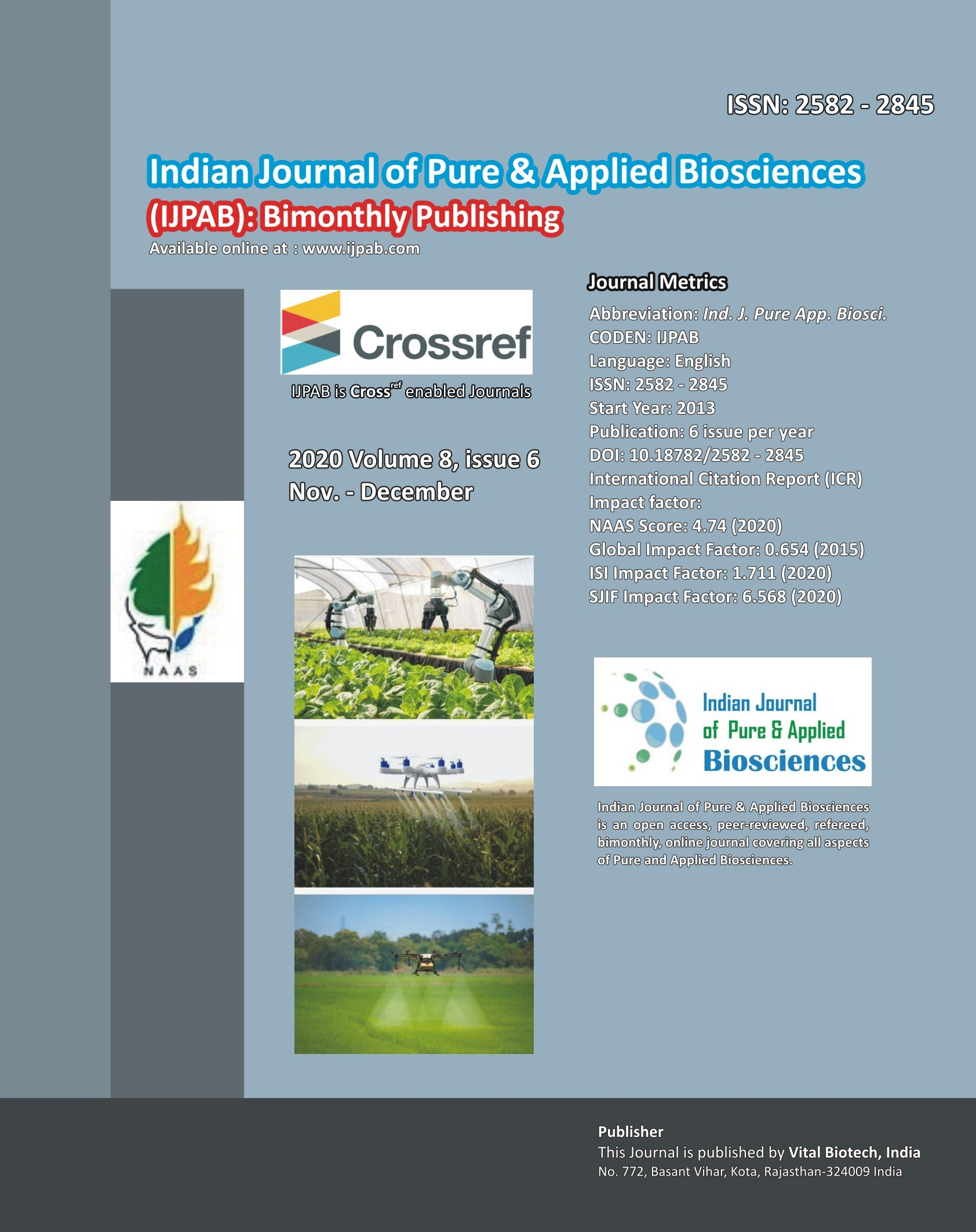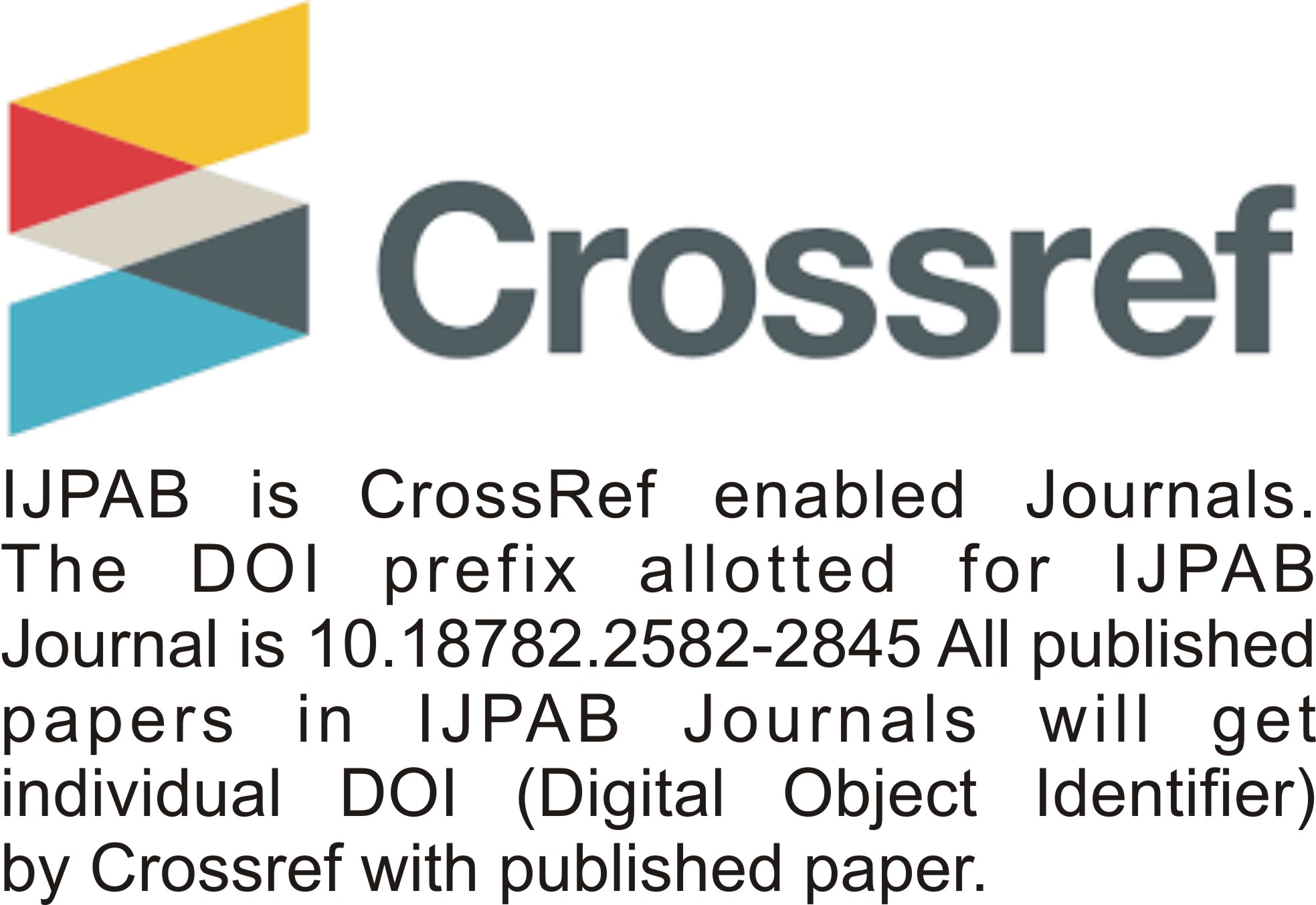INDIAN JOURNAL OF PURE & APPLIED BIOSCIENCES

ISSN (E) : 2582 – 2845

• ###### No. 772, Basant Vihar, Kota

Rajasthan-324009 India

• ###### Call Us On

+91 9784677044

• ###### Mail Us @

editor@ijpab.com

# Archives

Indian Journal of Pure & Applied Biosciences (IJPAB)
Year : 2020, Volume : 8, Issue : 6
First page : (523) Last page : (528)
Article doi: : http://dx.doi.org/10.18782/2582-2845.8488

Modified Estimation Using Different Linear Combination

Shabnum Gul*, P.P. Singh and Nisar Ahmad Khan
*Corresponding Author E-mail: khalidzara2018@gmail.com
Received: 15.11.2020 | Revised: 17.12.2020 | Accepted: 26.12.2020

ABSTRACT

The present study was taken under consideration in order to propose new modified estimators using different linear combinations for population mean using the auxiliary information of Mid-Range with coefficient of correlation, coefficient of variation and coefficient of skewness in order to achieve more precision in estimates than the already existing estimators. The properties associated with the proposed estimators are assessed by mean square error and bias and compared with the existing estimators. In the support of the theoretical proposed work we have given numerical illustration.

Keywords: Auxiliary information, Linear combinations estimators, Mean square error, Bias, Efficiency.

Full Text : PDF; Journal doi : http://dx.doi.org/10.18782

Cite this article: Gul, S., Singh, P. P., & Khan, N. A. (2020). Modified Estimation Using Different Linear Combination, Ind. J. Pure App. Biosci. 8(6), 523-528. doi: http://dx.doi.org/10.18782/2582-2845.8488

INTRODUCTION

An important purpose of sampling theory is to make sampling more efficient. It attempts to develop methods of sample selection and of estimation that provides, at the lowest possible cost, estimates that are precise enough for our purpose. This principle of specified precision at minimum cost recurs repeatedly in the presentation of theory. Estimation theory is an important part of statistical studies, whereby, population parameters are obtained using sample statistics. In any survey work, the experimenter’s interest is to make use of methods that will improve precisions of estimates of the population parameters both at the design stage and estimation stage. These parameters can be totals, means or proportions of some desired characters. In sample surveys, auxiliary information is used at selection as well as estimation stages to improve the design as well as obtaining more efficient estimators. Increased precision can be obtained when study variable Y is highly correlated with auxiliary variable X. Usually, in a class of efficient estimators, the estimator with minimum variance or mean square error is regarded as the most efficient estimator. Linear combination estimators are good examples in this context. Cochran (1940) initiated the use of auxiliary information at estimation stage and proposed ratio estimator for population mean. It is well established fact that linear combination estimators provide better efficiency in comparison to simple mean estimator if the study variable and auxiliary variable are positively correlated.

If the correlation between the study variable and auxiliary variables negative, product estimator given by Robson (1957) is more efficient than simple mean estimator.
Further improvements are also achieved on the classical linear combination by introducing a large number of modified linear combinatiob with the use of known parameters like, coefficient of variation, coefficient of kurtosis, coefficient of skewness and population correlation coefficient. For more detailed discussion one may refer to  Kadilar and Cingi (2004, 2006), Koyuncu and Kadilar (2009), Murthy (1967), Prasad (1989), Rao (1991), Singh (2003), Singh and Tailor (2003, 2005), Singh et al (2004), Sisodia and Dwivedi (1981), Upadhyaya and Singh (1999) and Yan and Tian (2010).
Further, had taken initiative by proposed modified linear combinations estimator for estimating the population mean of the study variable by using the population median of the auxiliary variable.

The objective of the paper is to propose modified estimators for estimating the population mean by using their linear combinations with the correlation coefficient and the coefficient of skewness of the auxiliary variable.

Table 3: The mean squared errors and Bias of the existing and proposed estimators

 Estimators MSE Bias T0; Kadilar and Cingi (2004) 2.317192 0.974223 T1;Yan and Tian (2010) 60.5325 14.60269 T2; Yan and Tian (2010) 35.18871 10.99178 T3; Kadilar and Cingi (2006) 53.98248 13.76056 T4; Kadilar and Cingi (2006) 52.63652 13.58105 T5; Kadilar and Cingi (2006) 50.78761 13.33051 T6; Kadilar and Cingi (2006) 42.40512 12.12991 Proposed 1 0.855269351 0.332265112 Proposed 2 0.855269334 0.312863365 Proposed 3 0.855269328 0.257737132

CONCLUSION

In this paper we have proposed modified estimators based on simple random sampling without replacement by using the auxiliary variable, under the situation when mid-range, coefficient of skewness and correlation coefficient is known. We found that the performances of our proposed estimators in terms of mean square error are more efficient than all other existing estimators in the literature. Hence we strongly recommend that our proposed estimators preferred over the existing estimators for use in practical application.

REFERENCES

Cochran, W. G. (1977). Sampling Techniques, Third Edition, Wiley Eastern Limited.
Cochran, W. G. (1940). The estimation of the yields of the cereal experiments by sampling for the ratio of grain to total produce, Journal of Agricultural Science, 30, 262-275.
Kadilar, C., & Cingi, H. (2006). An Improvement in Estimating the Population mean by using the Correlation Coefficient, Hacettepe Journal of Mathematics and Statistics, 35(1), 103-109.
Kadilar, C., & Cingi, H. (2004). Ratio estimators in simple random sampling, Applied Mathematics and Computation, 151, 893-902.
Koyuncu, N., & Kadilar, C. (2009). Efficient Estimators for the Population mean, Hacettepe Journal of Mathematics and Statistics, 38(2), 217-225.
Murthy, M. N. (1967). Sampling theory and methods, Statistical Publishing Society, Calcutta, India.
Prasad, B. (1989). Some improved ratio type estimators of population mean and ratio in finite population sample surveys, Communications in Statistics: Theory and Methods, 18, 379–392.
Rao, T. J. (1991). On certain methods of improving ratio and regression estimators, Communications in Statistics: Theory and Methods, 20(10), 3325–3340.
Robson, D. S. (1957). Application of multivariate Polykays to the theory of unbiased ratio type estimation, Journal of American Statistical Association, 52, 411-422.
Sisodia, B. V. S., & Dwivedi, V. K. (1981). A modified ratio estimator using coefficient of variation of auxiliary variable, Journal of the Indian Society of Agricultural Statistics, 33(1), 13-18.
Singh, H. P., & Tailor, R. (2005). Estimation of finite population mean with known coefficient of variation of an auxiliary, STATISTICA, anno LXV, 3, 301-31.
Singhand, H. P., & Tailor, R. (2003). Use of known correlation coefficient in estimating the finite population means, Statistics in Transition – new series, 6(4), 555-560.
Singh, H. P., Tailor, R., Tailor, R., & Kakran, M. S. (2004). An Improved Estimator of population means using Power transformation, Journal of the Indian Society of Agricultural Statistics, 58(2), 223-230.
Singh, D., & Chaudhary, F. S. (1986). Theory and Analysis of Sample Survey Designs, New Age International Publisher.
Singh, G. N. (2003). On the improvement of product method of estimation in sample surveys, Journal of the Indian Society of Agricultural Statistics, 56(3), 267–265.Subramani, J. (2013). A New Median Based Ratio Estimator for Estimation of the Finite Population Mean, Statistics- in Transition– Accepted for publication.

Upadhyaya, L. N., & Singh, H. P. (1999). Use of transformed auxiliary variable in estimating the finite population means, Biometrical Journal, 41(5), 627-636.